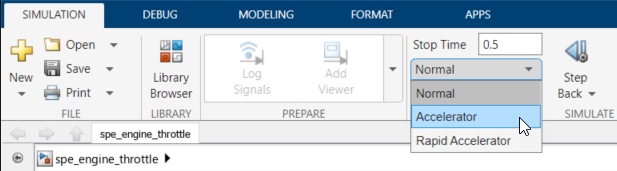Documentation

## Use Accelerator Mode During Simulations

Simulink® Design Optimization™ software supports `Normal` and `Accelerator` simulation modes. You can accelerate the design optimization computations by changing the simulation mode of your Simulink model to `Accelerator`. For information about these modes, see How Acceleration Modes Work (Simulink).

The default simulation mode is `Normal`. In this mode, Simulink uses interpreted code, rather than compiled C code during simulations.

In the `Accelerator` mode, Simulink Design Optimization software runs simulations during optimization with compiled C code. Using compiled C code speeds up the simulations and reduces the time to optimize the model response signals.

### Limitations

If the model structure changes during optimization, the model is compiled to regenerate the C code for each iteration. In this case, using the `Accelerator` mode increases the computation time. To learn more about code regeneration, see Code Regeneration in Accelerated Models (Simulink).

### Setting Accelerator Mode

To set the simulation mode to `Accelerator`, open the Simulink model window and perform one of the following actions:

• Under Simulation, choose `Accelerator` from the drop-down list as shown in the next figure.### Tip

To obtain the maximum performance from the `Accelerator` mode, close all Scope blocks in your model.

## Related Topics